# shapes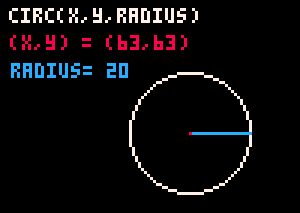`` circ( x, y, radius, color )``

circ = circle

 `x` number of pixels from the left going right `y` number of pixels from the top going down `radius` number of pixels from the center to the outside `color` (optional) a color number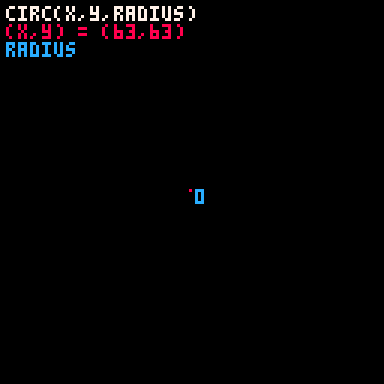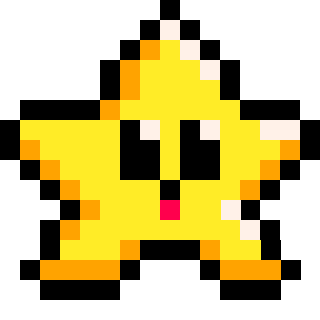`` circfill( x, y, radius, color )``

circfill = circle filled

 `x` number of pixels from the left going right `y` number of pixels from the top going down `radius` number of pixels from the center to the outside `color` (optional) a color number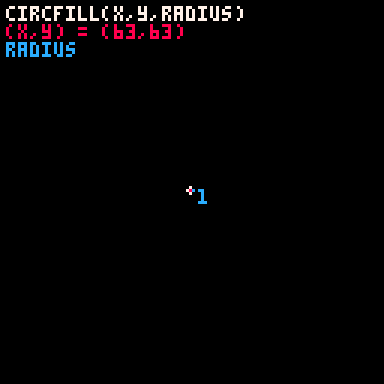320

20 Mar 2023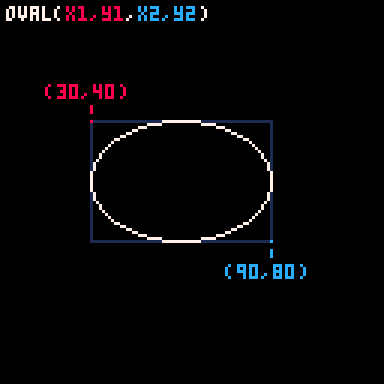`` oval( x1, y1, x2, y2, color )``

 `x1` number of pixels from the left going right for the first corner `y1` number of pixels from the top going down for the first corner `x2` number of pixels from the left going right for the second corner `y2` number of pixels from the top going down for the second corner `color` (optional) a color number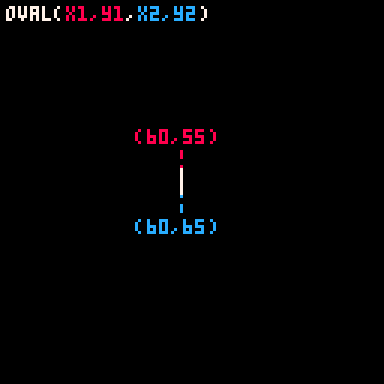`` ovalfill( x1, y1, x2, y2, color )``

 `x1` number of pixels from the left going right for the first corner `y1` number of pixels from the top going down for the first corner `x2` number of pixels from the left going right for the second corner `y2` number of pixels from the top going down for the second corner `color` (optional) a color number281

20 Mar 2023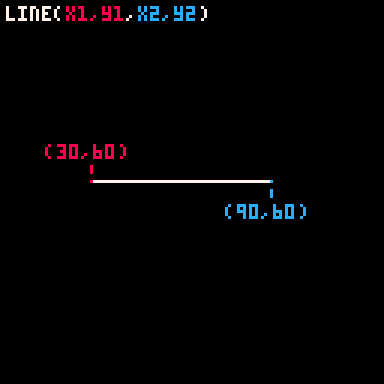`` line( x1, y1, x2, y2, color )``

 `x1` number of pixels from the left going right for the starting point `y1` number of pixels from the top going down for the starting point `x2` number of pixels from the left going right for the end point `y2` number of pixels from the top going down for the end point `color` (optional) a color number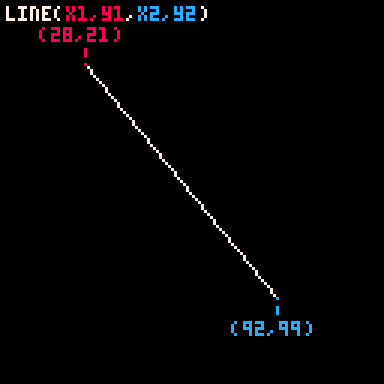286

20 Mar 2023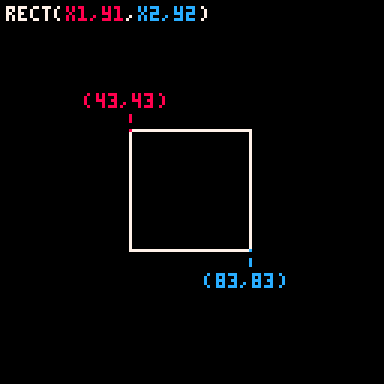`` rect( x1, y1, x2, y2, color )``

rect = rectangle

 `x1` number of pixels from the left going right for the first corner `y1` number of pixels from the top going down for the first corner `x2` number of pixels from the left going right for the second corner `y2` number of pixels from the top going down for the second corner `color` (optional) a color number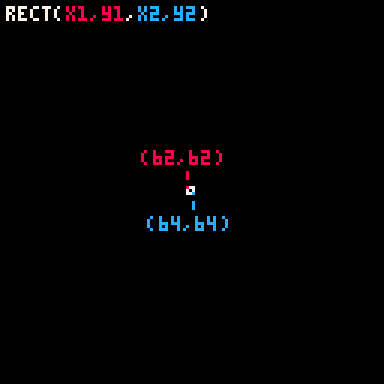`` rectfill( x1, y1, x2, y2, color )``

rectfill = rectangle filled

 `x1` number of pixels from the left going right for the first corner `y1` number of pixels from the top going down for the first corner `x2` number of pixels from the left going right for the second corner `y2` number of pixels from the top going down for the second corner `color` (optional) a color number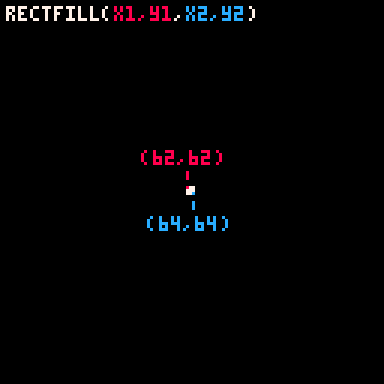321

21 Mar 2023

fillp = fill pattern

`` fillp( p )``
 `p` a number value (-32768 to 32768) that sets the pattern

`fillp(P)` is a PICO-8 lua function that sets a 4x4 2-color pattern that is tiled when drawing shape functions to the screen. Functions such as: `circ`, `circfill`, `rect`, `rectfill`, `oval`, `ovalfill`, `pset`, and `line`.

#### Custom Fill Pattern

The 4x4 pixel grid used by `fillp` looks like this; with each pixel designated by a value: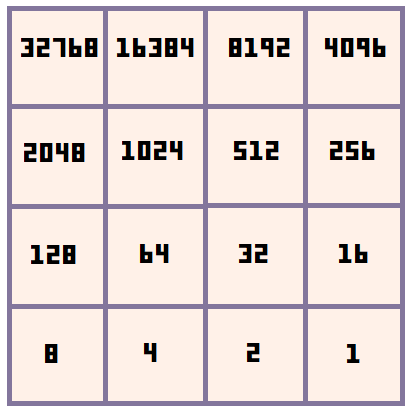The pattern set by `fillp()` is specified by the value of the argument ( `P` used here as an example ), which is a bitfield. The default value is 0, so `fillp()` or `fillp(0)` both reset the pattern to a full solid fill pattern. Then if we set the value to 1, then it will flip the bottom right pixel.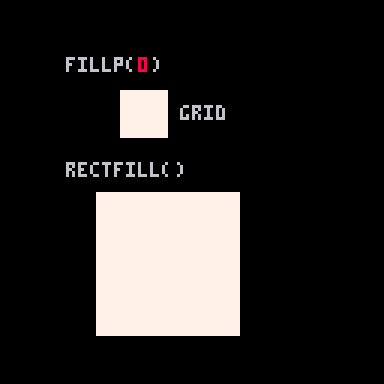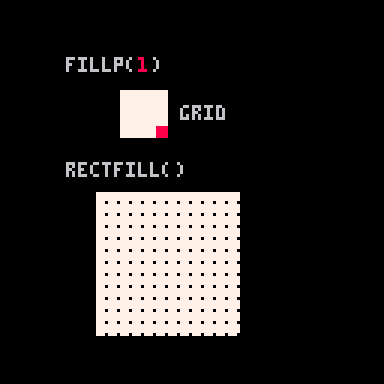If we continue adding just 1 to the value, then you'll see that a value of 2 will flip the second pixel on the bottom right, but not the bottom right corner anymore. Refer back to the grid above to understand how each pixel in the grid has a value.

Continuing on, a value of 3 will flip both the first and second pixels on the bottom right because 3 is 1 and 2: ( 3 = 1 + 2 ).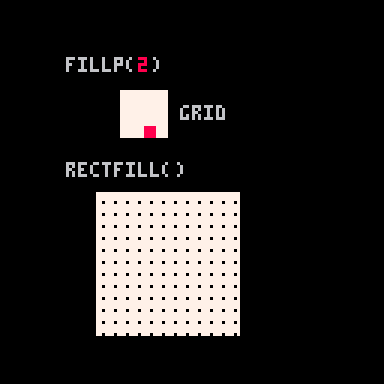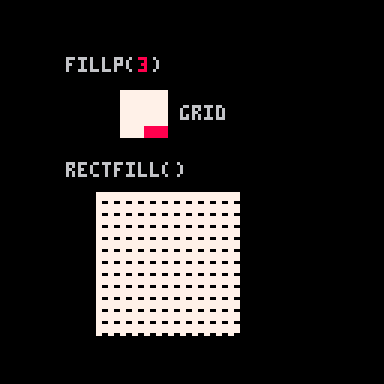Just like we added the bit values of 1 and 2 to get the pattern value of 3, you can find the exact pattern you want by simply adding together the bit values. For example:

``fillp(2+8+16+64) --90``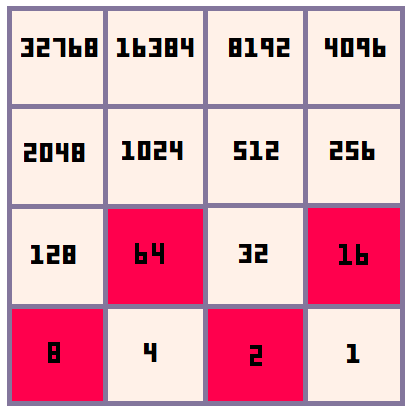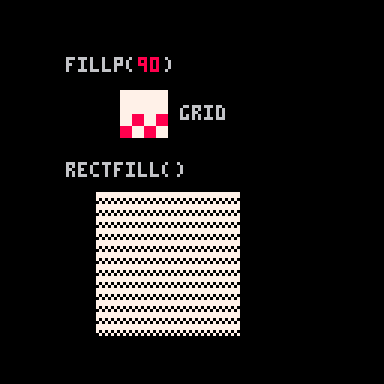Here are some more example fill patterns: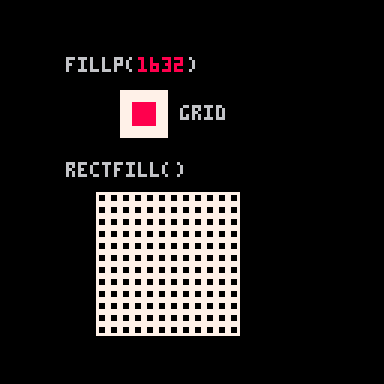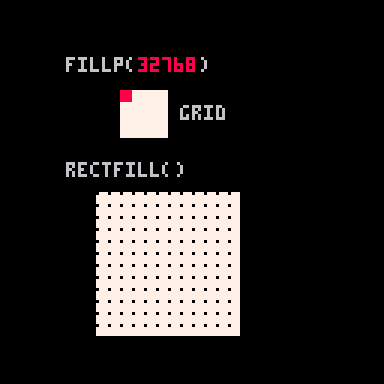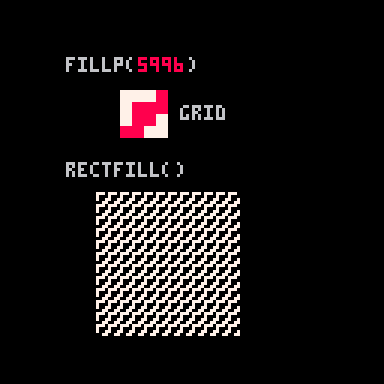For example, `fillp(4+8+64+128+256+512+4096+8192)` would produce a checkerboard pattern, which can also be expressed in binary as fillp(0b0011001111001100).

``````fillp(4+8+64+128+256+512+4096+8192)
fillp(0b0011001111001100)``````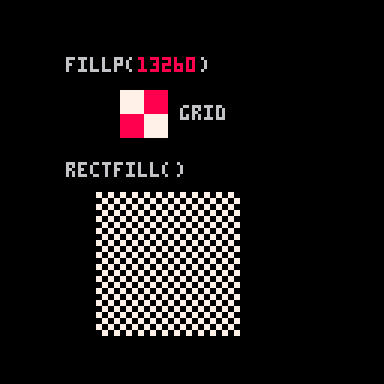#### All Possible Patterns

Zep created a cart that displays all 433 unique possible patterns (any duplicate patterns that are inverted, rotated, flipped, or shifted have been ignored). Try it on the BBS here: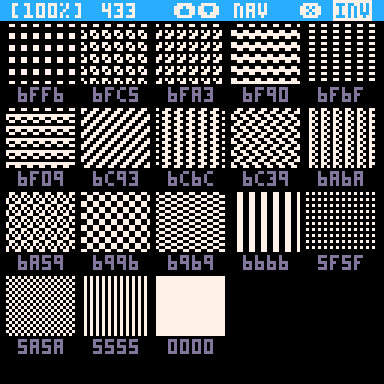To load this cart directly in your PICO-8 (also Education Edition) use the command `load #fillp_cat` and press enter.To be added: Further explanation for setting a second color using "high bits", manual example: [ CIRCFILL(64,64,20, 0x4E) -- brown and pink ] and how to set fillp to apply transparency, or to sprites, and secondary palette. See the manual for more information.540

3 Nov 2023

`Font`